boundary

Boundary of a set of points in 2-D or 3-D

Description

example

k = boundary(x,y) returns a vector of point indices representing a single conforming 2-D boundary around the points (x,y). The points (x(k),y(k)) form the boundary. Unlike the convex hull, the boundary can shrink towards the interior of the hull to envelop the points.

example

k = boundary(x,y,z) returns a triangulation representing a single conforming 3-D boundary around the points (x,y,z). Each row of k is a triangle defined in terms of the point indices.

example

k = boundary(P) specifies points (x,y) or (x,y,z) in the columns of matrix P.

example

k = boundary(___,s) specifies shrink factor s using any of the previous syntaxes. s is a scalar between 0 and 1. Setting s to 0 gives the convex hull, and setting s to 1 gives a compact boundary that envelops the points. The default shrink factor is 0.5.

example

[k,v] = boundary(___) also returns a scalar v, which is the area (2-D) or volume (3-D) which boundary k encloses.

Examples

collapse all

Create and plot a set of random 2-D points.

rng('default')
x = rand(30,1);
y = rand(30,1);
plot(x,y,'.')
xlim([-0.2 1.2])
ylim([-0.2 1.2])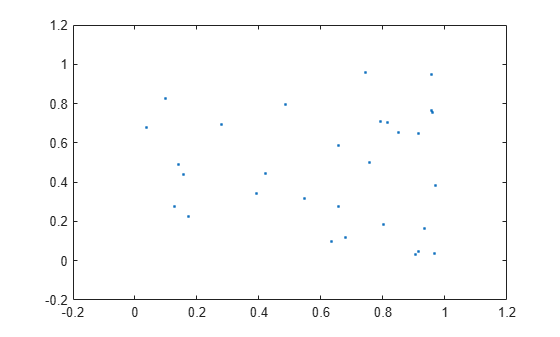Compute a boundary around the points using the default shrink factor.

k = boundary(x,y);
hold on;
plot(x(k),y(k));Create a new boundary around the points using a shrink factor of 0.1. The result is a less compact boundary enveloping the points.

j = boundary(x,y,0.1);
hold on;
plot(x(j),y(j));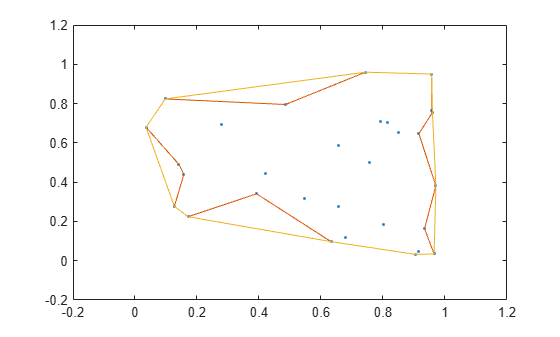Create and plot a set of random 3-D points.

rng('default')
P = rand(30,3);
plot3(P(:,1),P(:,2),P(:,3),'.','MarkerSize',10)
grid on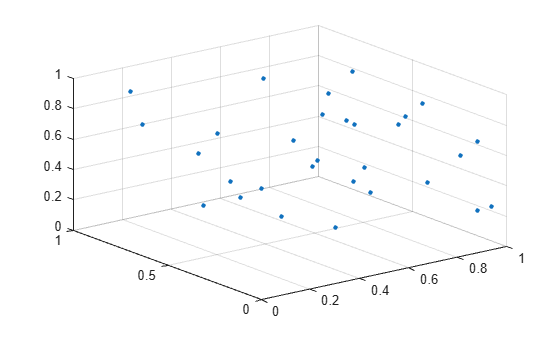Plot the boundary using the default shrink factor.

k = boundary(P);
hold on
trisurf(k,P(:,1),P(:,2),P(:,3),'Facecolor','red','FaceAlpha',0.1)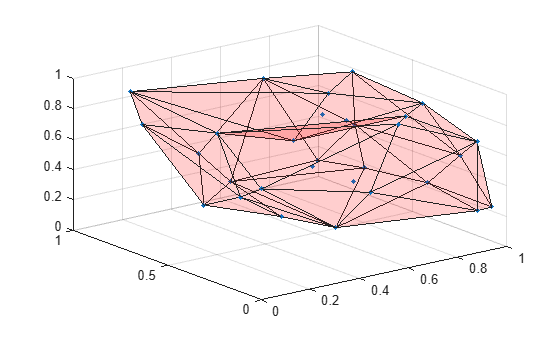Create and plot a set of random 3-D points.

rng default;
P = rand(30,3);
plot3(P(:,1),P(:,2),P(:,3),'.')
grid onCompute two boundaries: one with a shrink factor of 0 and the other with a shrink factor of 1.

k = boundary(P,0);
j = boundary(P,1);

Compare the shrink factors by plotting the original points and the two boundaries side-by-side.

subplot(1,2,1);
plot3(P(:,1),P(:,2),P(:,3),'.','MarkerSize',10)
hold on
trisurf(k,P(:,1),P(:,2),P(:,3),'FaceColor','red','FaceAlpha',0.1)
axis equal
title('Shrink Factor = 0')

subplot(1,2,2);
plot3(P(:,1),P(:,2),P(:,3),'.','MarkerSize',10)
hold on
trisurf(j,P(:,1),P(:,2),P(:,3),'FaceColor','red','FaceAlpha',0.1)
axis equal
title('Shrink Factor = 1')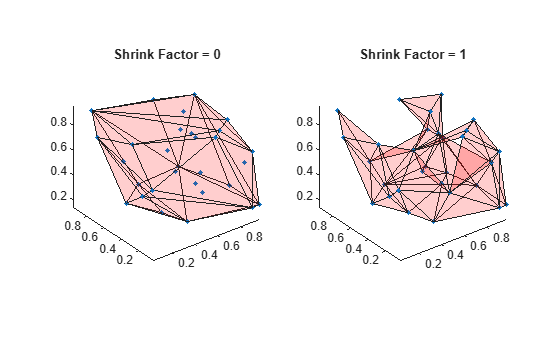Create and plot a set of random 3-D points.

rng('default')
P = rand(30,3);
plot3(P(:,1),P(:,2),P(:,3),'.')
grid on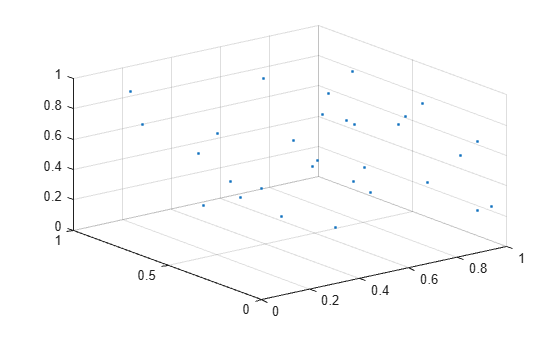Use the boundary function to compute a boundary around the points, and find the volume of the resulting shape.

[~, vol] = boundary(P);
vol
vol = 0.2962

Input Arguments

collapse all

x-coordinates of points, specified as a column vector.

Data Types: double

y-coordinates of points, specified as a column vector.

Data Types: double

z-coordinates of points, specified as a column vector.

Data Types: double

Point coordinates, specified as a matrix with two columns (for a 2-D alpha shape) or a matrix with three columns (for a 3-D alpha shape).

• For 2-D, the columns of P represent x and y coordinates, respectively.

• For 3-D, the columns of P represent x, y, and z coordinates, respectively.

Data Types: double

Shrink factor, specified as a scalar in the range of [0,1].

• s = 0 corresponds to the convex hull of the points.

• s = 1 corresponds to the tightest single-region boundary around the points.

The default shrink factor is 0.5. Specify a larger or smaller shrink factor to tighten or loosen the boundary around the points, respectively.

Example: k = boundary(x,y,0.76) specifies a shrink factor of 0.76, producing a tighter boundary than the default.

Output Arguments

collapse all

Boundary point indices, returned as a vector or matrix. k contains the indices of the input points that lie on the boundary.

• For 2-D problems, k is a column vector of point indices representing the sequence of points around the boundary, which is a polygon.

• For 3-D problems, k is a triangulation matrix of size mtri-by-3, where mtri is the number of triangular facets on the boundary. Each row of k defines a triangle in terms of the point indices, and the triangles collectively form a bounding polyhedron.

Area or volume enclosed by boundary, returned as a scalar.

• For 2-D problems, v is the area enclosed by boundary k.

• For 3-D problems, v is the volume enclosed by boundary k.

Algorithms

boundary constructs an alphaShape from the specified points and then uses boundaryFacets to determine which points lie on the boundary.